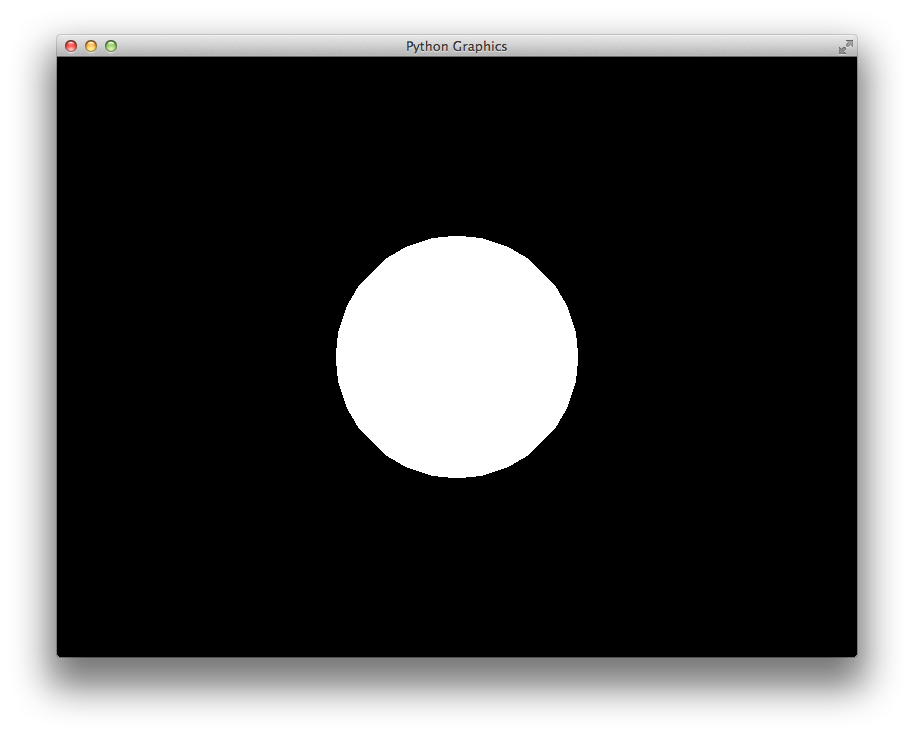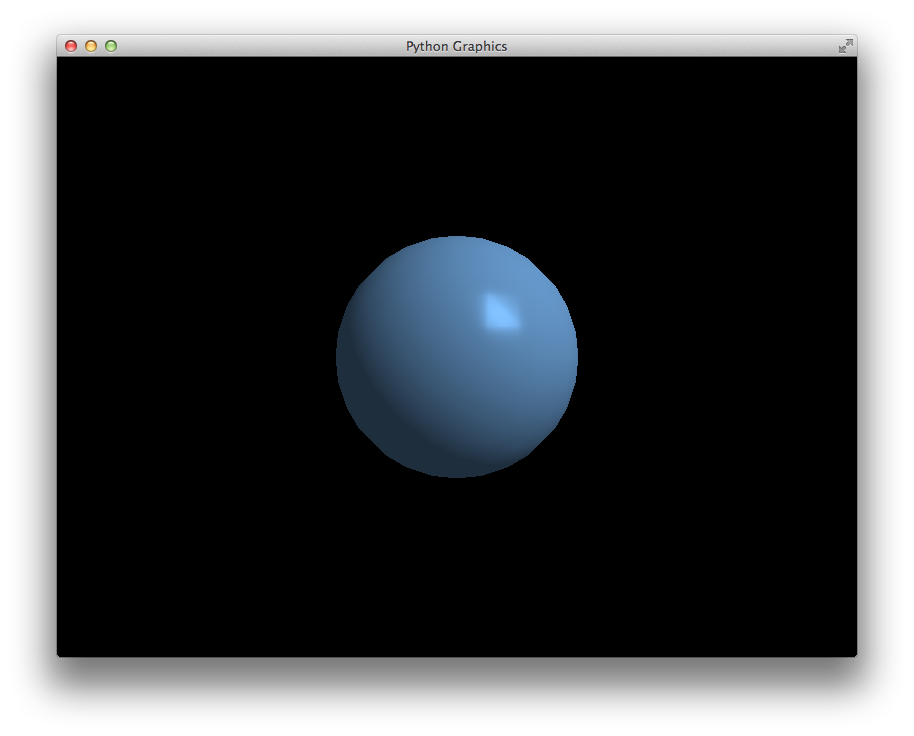# Tutorial¶

In this tutorial we will explore various aspects of the `pg` framework from opening a window to flying through a scene of several geometric objects.

## Creating a Window¶

The first step in any `pg` program is to create an `App` object. This object owns the main window loop. Then you can instantiate one or more `Window` objects:

```import pg

app = pg.App()
pg.Window()
app.run()
```

This will create your first blank window. However, typically we will want to extend the `Window` class to add our own functionality. We should also only construct and run the `App` inside of a `__main__` block:

```import pg

class Window(pg.Window):
pass

if __name__ == "__main__":
app = pg.App()
Window()
app.run()
```

This `__main__` block is so common that `pg` includes a shortcut:

```if __name__ == "__main__":
pg.run(Window)
```

Note that the `run` function takes a `Window` class, not an instance.

## Window Lifecycle¶

Typically we will override several methods in the `Window` class. Together, these methods manage the lifecycle of the `Window`.

• `setup()`: code to be run once when the window is created
• `update(t, dt)`: called each frame with elapsed time and time since last frame
• `draw()`: called each frame for rendering the scene
• `teardown()`: cleanup to be performed when the window is closed

Here is a basic code template for starting a new `pg` project:

```import pg

class Window(pg.Window):
def setup(self):
pass
def update(self, t, dt):
pass
def draw(self):
pass
def teardown(self):
pass

if __name__ == "__main__":
pg.run(Window)
```

Modern OpenGL uses shaders and programs in place of the deprecated, fixed-function pipeline. `pg` provides classes to easily work with shaders and even includes some built-in shaders with basic functionality.

In our `Window.setup` function we should load and compile a shader program that will render our geometric primitives. One of the built-in shaders is `SolidColorProgram` which renders all primitives with the specified color:

```self.program = pg.SolidColorProgram()
```

To hold the configuration for our program, we must use a `Context`. Multiple contexts can be created for a single program:

```self.context = pg.Context(self.program)
```

Now, we can set attributes on the context corresponding to the attributes and uniforms defined in the vertex and fragment shaders. The `SolidColorProgram` defines a `color` uniform. Let’s set it to white:

```self.context.color = (1, 1, 1)
```

If you wanted to use your own vertex and fragment shaders, you would simply do the following instead:

```self.program = pg.Program(vs, fs)
```

`vs` and `fs` can be the shader source code or a filename or instances of `VertexShader` and `FragmentShader`, respectively.

Our `setup` function is not yet complete but looks like this:

```def setup(self):
self.program = pg.SolidColorProgram()
self.context = pg.Context(self.program)
self.context.color = (1, 1, 1)
```

## Built-in Geometric Shapes¶

`pg` includes functions for generating several 3-dimensional primitives including spheres, cuboids, cylinders, cones, planes, axes, etc.

Let’s create a sphere:

```sphere = pg.Sphere(3, 0.5, (0, 0, 0))
```

The first argument, detail, indicates how detailed to make the sphere. It is the number of times to recursively split the triangles. The second argument specifies the radius and the third argument specifies the center of the sphere.

## Vertex Buffers¶

The sphere object has lists specifying its vertex positions, normals and texture coordinates. For the `SolidColorProgram`, we only need the positions.

Now it’s time to load this data into a vertex buffer so our graphics card can access it:

```self.context.position = pg.VertexBuffer(sphere.positions)
```

## Transformation Matrices¶

Dealing with matrices is a big part of using OpenGL. `pg` includes a `Matrix` class that will help us with most scenarios.

For our code, we’ll set the camera position with a translation and we’ll use a perspective projection:

```matrix = pg.Matrix()
matrix = matrix.translate((0, 0, -2))
matrix = matrix.perspective(65, self.aspect, 0.1, 100)
self.context.matrix = matrix
```

Now our setup function is complete:

```def setup(self):
self.program = pg.SolidColorProgram()
self.context = pg.Context(self.program)
self.context.color = (1, 1, 1)
sphere = pg.Sphere(3, 0.5, (0, 0, 0))
self.context.position = pg.VertexBuffer(sphere.positions)
matrix = pg.Matrix()
matrix = matrix.translate((0, 0, -2))
matrix = matrix.perspective(65, self.aspect, 0.1, 100)
self.context.matrix = matrix
```

## Rendering¶

Finally, we can render the scene as shown below:

```def draw(self):
self.clear()
self.context.draw(pg.GL_TRIANGLES)
```

Because we’re using a single color without shading, our sphere just looks like a circle right now.We can instead use the `DirectionalLightProgram` which renders the scene with a single, directional light source. This program has several uniforms that can be configured but most of them have sensible defaults. At a minimum we should set the `camera_position` so that the lighting will look correct:

```self.context.camera_position = (0, 0, 2)
```

We also now need to provide the sphere normal vectors to the program:

```self.context.normal = pg.VertexBuffer(sphere.normals)
```

Here is the updated code:

```class Window(pg.Window):
def setup(self):
self.program = pg.DirectionalLightProgram()
self.context = pg.Context(self.program)
sphere = pg.Sphere(3, 0.5, (0, 0, 0))
self.context.position = pg.VertexBuffer(sphere.positions)
self.context.normal = pg.VertexBuffer(sphere.normals)
matrix = pg.Matrix()
matrix = matrix.translate((0, 0, -2))
matrix = matrix.perspective(65, self.aspect, 0.1, 100)
self.context.matrix = matrix
self.context.camera_position = (0, 0, 2)
def draw(self):
self.clear()
self.context.draw(pg.GL_TRIANGLES)
```

And here is what it looks like.## Flying Around with WASD¶

`pg` includes a `WASD` class that makes it incredibly easy to fly around your scene. The `WASD` object hooks into your window’s keyboard and mouse callbacks and provides you with a matrix with the translation and rotation for the camera position.

First, let’s construct the `WASD` object in our `setup` function:

```self.wasd = pg.WASD(self)
```

The initial camera position and viewing target can be set with `WASD.look_at`:

```self.wasd.look_at((0, 0, 2), (0, 0, 0))
```

Now we need to update our context’s matrix each frame. The matrix code is removed from the `setup` function and goes in the `update` function with a few changes:

```def update(self, t, dt):
matrix = self.wasd.get_matrix()
matrix = matrix.perspective(65, self.aspect, 0.1, 100)
self.context.matrix = matrix
self.context.camera_position = self.wasd.position
```

## Complete Example¶

```import pg

class Window(pg.Window):
def setup(self):
self.wasd = pg.WASD(self)
self.wasd.look_at((0, 0, 2), (0, 0, 0))
self.program = pg.DirectionalLightProgram()
self.context = pg.Context(self.program)
sphere = pg.Sphere(3, 0.5, (0, 0, 0))
self.context.position = pg.VertexBuffer(sphere.positions)
self.context.normal = pg.VertexBuffer(sphere.normals)
def update(self, t, dt):
matrix = self.wasd.get_matrix()
matrix = matrix.perspective(65, self.aspect, 0.1, 100)
self.context.matrix = matrix
self.context.camera_position = self.wasd.position
def draw(self):
self.clear()
self.context.draw(pg.GL_TRIANGLES)

if __name__ == "__main__":
pg.run(Window)
```